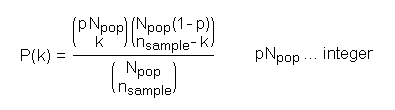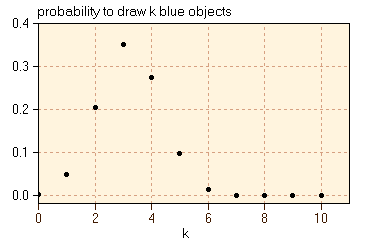The SDL Component Suite is an industry leading collection of components supporting scientific and engineering computing. Please visit the SDL Web site for more information....## HyperGeoDistriDensity

 Unit: SDL_statis Class: none Declaration: function HyperGeoDistriDensity (p: double; npop, nsample, k: integer): double;

The function HyperGeoDistriDensity returns the density value of the hypergeometric distribution. The parameter p denotes the probability of the occurrence of an interesting case. The parameter npop specifies the total number of cases. The parameter nsample specifies the number of observations drawn from the population of npop cases. Finally, the parameter k defines the number of interesting cases to be expected.The product p times npop must be an integer and equals the number of interesting cases in the population.

The following figure shows the probability distribution for an experiment with a total of 20 objects, 8 of these being of blue color. The points show the probability to draw exactly k blue objects if the sample size is 8.For this example the parameters of the hypergeometric distribution have the following values:

p = 0.4
npop = 20
nsample = 8
k = 0..10

 Hint 1: Please note that the hypergeometric distribution is calculated by using the logarithm of the gamma function (LnGamma) which is an approximation. Thus the results of HyperGeoDistriDensity are also approximations, being accurate to only about 5 to 8 decimal places.

 Hint 2: Background information on the hypergeometric distribution can be found in Fundamentals of Statistics.

Last Update: 2020-Aug-12